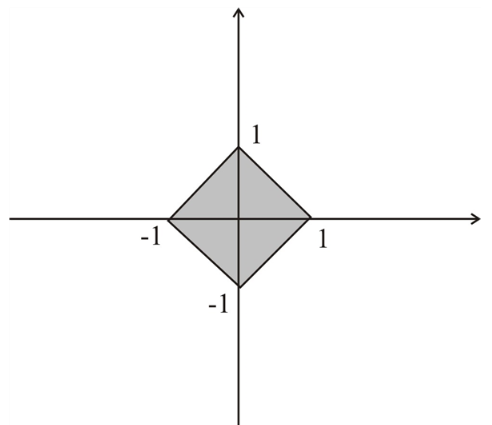# The sketch for the region in the plane that consist of all the points ( x , y ) such that | x | + | y | ≤ 1 .### Single Variable Calculus: Concepts...

4th Edition
James Stewart
Publisher: Cengage Learning
ISBN: 9781337687805### Single Variable Calculus: Concepts...

4th Edition
James Stewart
Publisher: Cengage Learning
ISBN: 9781337687805

#### Solutions

Chapter 1, Problem 9P
To determine

## To find: The sketch for the region in the plane that consist of all the points (x,y) such that |x|+|y|≤1 .

Expert Solution

### Explanation of Solution

Given:

The given condition is |x|+|y|1 .

Calculation:

Consider the given condition is,

|x|+|y|1

Consider the case when x>0 and y>0 in the second quadrant then by the condition of the modules of the function,

x+y=1

Consider the case when x<0 and y<0 in the second quadrant then by the condition of the modules of the function,

x+y=1

Consider the case when x<0 and y<0 in the second quadrant then by the condition of the modules of the function,

xy=1

Consider the case when x>0 and y<0 in the second quadrant then by the condition of the modules of the function,

xy=1

This shows that the available region on the graph are [1,1] and [1,1] , so the graph is the square shape region as shown in Figure 1Figure 1

### Have a homework question?

Subscribe to bartleby learn! Ask subject matter experts 30 homework questions each month. Plus, you’ll have access to millions of step-by-step textbook answers!